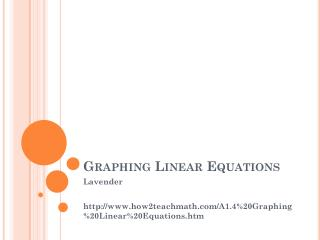DownloadDownload PresentationGraphing Linear Equations

# Graphing Linear Equations

Télécharger la présentation## Graphing Linear Equations

- - - - - - - - - - - - - - - - - - - - - - - - - - - E N D - - - - - - - - - - - - - - - - - - - - - - - - - - -
##### Presentation Transcript

1. Graphing Linear Equations Lavender http://www.how2teachmath.com/A1.4%20Graphing%20Linear%20Equations.htm

2. Familiar With a 4 quadrant grid? • Draw a 4 quadrant grid – Number each axis from -5 to 5 • DO NOT draw in the grid lines

3. Plotting Coordinates • Coordinates are the points on a line • ( X , Y ) • X meaning X axis • Y meaning Y axis • Plot the coordinates ( 5 , -3 ) on the grid you labeled. ( 5 , -3 )

4. Plot ALL of the following coordinates • On the grid you previously labeled • ( 3 , 3 ) • ( 2 , -5 ) • ( 1 , 0 ) • ( -4 , -2 ) • ( -1 , 1 ) • ( -2 , 5 ) • ( 4 , -1 )

5. Graphing from a TABLE

6. Graphing from a TABLE

7. Graphing from a TABLE

8. WARM-UP • SOLVE the following equations for X… • X − 10 = −3 • X/5 = 2 • X − 7 = −1 • −40 = −5X • 418 = −22a

9. Solve for X when Y=…. • Y = 3 x + 9 = y x = _________ • Y = 10 2x = y x = _________ • Y = 7 x/3 = y x = _________ • Y = 2 32 – x = y x = _________ • Y = 100 x2 = y x = _________

10. Graphing from T-CHARTS & Equations • Equation: Y = X

11. Graphing from T-CHARTS & Equations • Equation: Y = 3X - 2

12. Graphing from T-CHARTS & Equations • Equation: Y = -2X + 4

13. Graphing from T-CHARTS & Equations – inputs not given • Equation: Y = 3X

14. Graphing from T-CHARTS & Equations – inputs not given • Equation: Y = X - 2

15. Graphing from T-CHARTS & Equations – inputs not given • Equation: Y = -X + 4